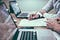Photo by Helloquence on Unsplash

Calculating fractions in python is very easy. There are build in Fractions functions to calculate all kind of Fractions. So my eldest son had some homework where he had to do some calculations with fractions. As he hates doing homework I always try to persuade him to do make his homework. So the other day I made a bet with him. I told him I could write a small script that would calculate any of this homework calculations within the time he needed to actually do the calculation. Python won :-)

As I have told calculations within python are very easy. Calculating fractions, a numerical quantity that is not a whole number, with the fractions package is even easier. The fractions module provides support for rational number arithmetic.

A fraction is a number that represents a whole number that has been divided into equal parts.

With the script below you can calculate easy fractions like:

`1/2 * 2/4or3/4 - 2/27or2/34 / 2/43or1/2 + 4/5`

As you can see you can put in 4 operators to do the calculation with. Add, Substract, divede and multiply.

Python operator is a symbol that performs an operation on one or more operands. An operand is a variable or a value on which we perform the operation.

Let me explain the script to you in parts.

## Fraction regex

So my script let’s you actually just type in the calculation you want to make.

But for getting out the fractions and the operator you need a regex. Well you can do it in any other way, but I use one simple regex.

`regex = r"([0-9]*\/[0-9*])(\+|\-|\*|\:|\/)([0-9]*\/[0-9*])"`

So this regex is devided in to 3 parts.

1. `([0-9]*\/[0-9*])`
2. `(\+|\-|\*|\:|\/)`
3. `([0-9]*\/[0-9*])`

So the first and last part are the same. They group number(s) / (slash) and number(s)

It gets out the first and last fraction used that is typed in by the user.

So the [0–9] tells the regex to search for 0 to 9 numbers and the * after it tells the regex to for 1 or more numbers. So you get [0–9]*

The `\/` tells to search for a / (slash) you have to put \ infront of it else the regex thinks it is just a regex operator.

The middle part `(\+|\-|\*|\:|\/)` tells the regex to search for either a + or - or * or : (in the netherlands we use : in stead of /) or /.

So it searches for an operator. The | is the OR operator and tells the regex OR plus OR minus OR… well you get the point.

So if you put this in the regex

`1/2+3/4`

The first part will find : 1/2 The second part will find : + and the third part will find : 3/4

If you put this in the regex

`a/a+3/4`

It will find nothing as it breaks the first part of the regex.

So if you put spaces between the operator, it will also break.

That is why I use

`frac = frac.replace(" ",'')`

in my script just before I put the string in the regex. It will remove all found (white)spaces so the regex will work.

So this:

`1/2 + 3/4`

Will be set to

`1/2+3/4`

## Operator in python

So the second part I want to tell you about is the operator part.

In my regex you can only use Add, Subtract, Divide and Multiply.

If you use the Operation module (or package) you can well Add, Subtract, Divide and Multiply very easily.

If you want to add to numbers, you can either do:

`1 + 3`

or:

`operator.add(1, 3)`

As you can see, the operator usage is much longer than the add sign. But I have to use this because from in input python does not really understand what a plus or minus sign is.

So I’m using this part of the script

`ops = {'+': operator.add,       '-': operator.sub,       '/': operator.truediv,       ':': operator.truediv,       '*': operator.mul}`

To setup the kinds of operators I want to use.

And by calling upon the Ops dictinary with the key like:

`ops['+']`

I’m actually telling to use the operator.add function

Are you still with me?

## Fractions in Python

This module provides support for rational number arithmetic. It allows to create a Fraction instance from integers, floats, numbers, decimals and strings.

So by using the Fractions module you can simplify fractions and calculate with them.

a simple

`from fractions import Fractionprint(Fraction(2/4))`

will result in

`1/2`

And a simple

`from fractions import Fractionprint(Fraction(1/2)-Fraction(6/12))`

Will result in

`0`

Yes, zero… the second fraction 6/12 is actually 1/2 and 1/2 minus 1/2 is Yes, Zero :-)

Combining it all will result in the code below.

Have fun! And don’t forget! Doing homework by yourself is very important! So don’t use this python script to solve you Fraction problems.

Written by

## purepython

#### Scribbles of code in Python. A new Medium publication about Python coding and other Tech.

Welcome to a place where words matter. On Medium, smart voices and original ideas take center stage - with no ads in sight. Watch
Follow all the topics you care about, and we’ll deliver the best stories for you to your homepage and inbox. Explore
Get unlimited access to the best stories on Medium — and support writers while you’re at it. Just \$5/month. Upgrade